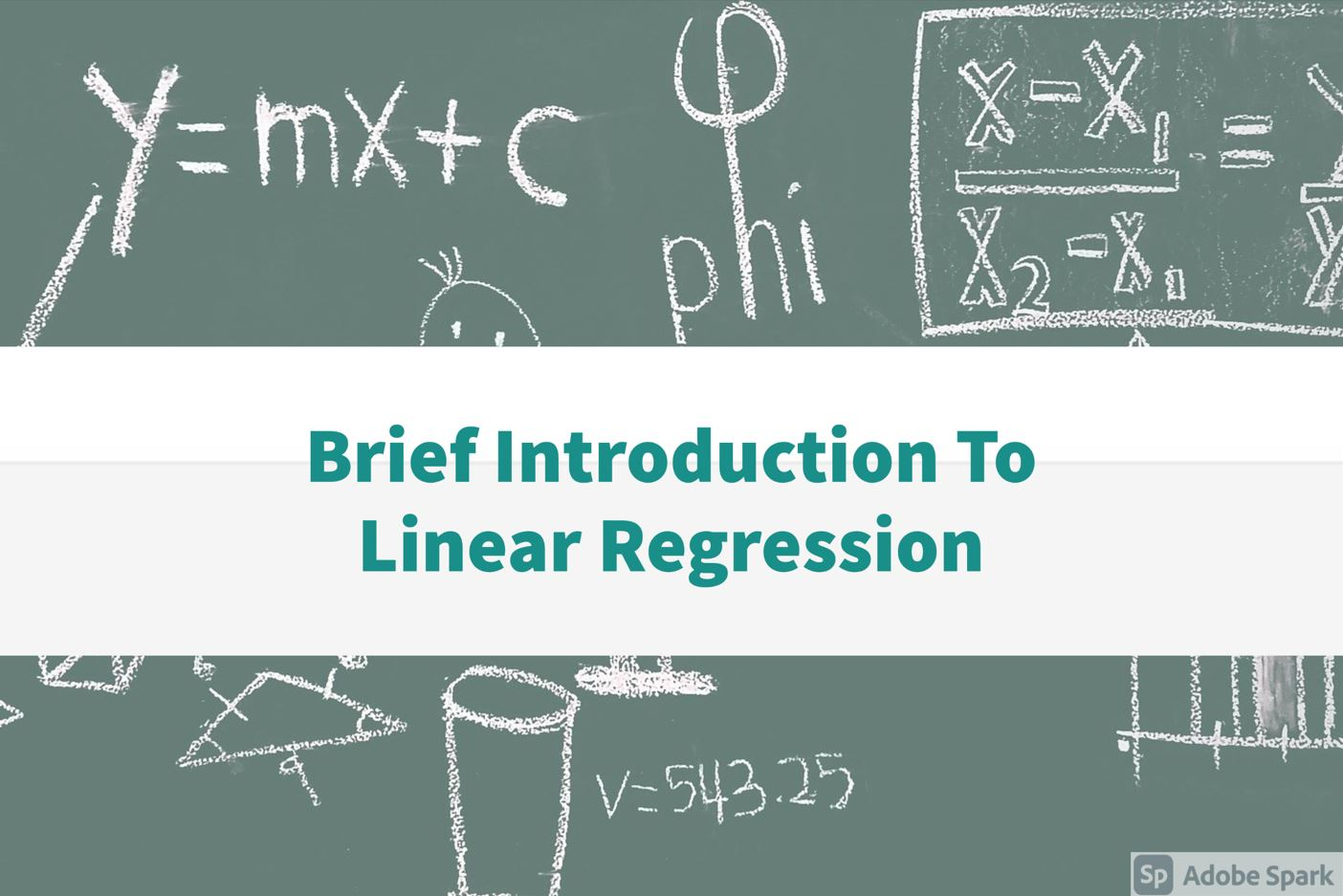All

### Lets see how we can add two numbers in python.

for this topic if you are unfimiliar with python variables, please give 1 minute to this page

pythonocean.com/watch-course/variables-in-python

first declare two variables

``````
a = 2
b = 3
```
```

now a has value 2 and and b has value 3

make another variable result and

``````
result = a+b
#Now print this result
```
```

Complete code

``````
a = 3
b = 2
result = a+b
```
```

To get input from user use input() method

``````

a = float(input("Enter first number :"))
b = float(input("Enter second number :"))

result = a+b
print(result)

```
```

### Subscribe to my youtube channel

AllBest Programming laptops to buy in 2021

May 23, 2021, 12:19 p.m.#### Start watching python tutorialsPython Essentials

April 21, 2021, 6:08 p.m.